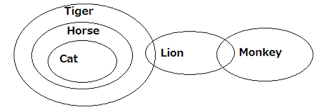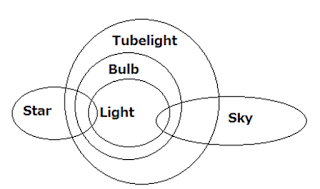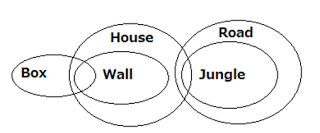Reasoning Practice Quiz for IBPS Clerk (Set - 4)

Reasoning Practice Quiz for IBPS Clerk (Set - 4)
Directions (1 – 5): Study the given information and answer the questions:
A word and number arrangement machine when given an input line of words and numbers rearranges them following a particular rule in each step. The following is an illustration of an input and rearrangement.
Input:  they 68 also 59 called 76 for 33 greater 46 strength 91
Step I:  also they 68 59 called 76 for greater 46 strength 91 34
Step II: called also they 68 76 for greater 46 strength 91 34 60
Step III:  for called also they 68 76 greater 46 strength 34 60 92
Step IV:  greater for called also they 68 76 strength 34 60 92 45
Step V: strength greater for called also they 76 34 60 92 45 67
Step VI: they strength greater for called also 34 60 92 45 67 75
And Step VI is the last step of the rearrangement of the above input.
As per the rules followed in the above steps, find out in each of the following questions the appropriate step for the given input.
Input:  because 56 such 29 act 24 put 17 extreme 32 risk 33
1. In which step the elements ‘56 such 18’ found in the same order?
(a) Step I
(b) Step II
(c) Step III
(d) Step V
(e)Step VI
2. In step IV, which of the following words would be at 3rd position to the left of 5th from the right end?
(a) act
(b) such
(c) because
(d) 56
(e) extreme
3. How many step required completing the above arrangement?
(a) Three
(b) Four
(c) Six
(d) Seven
(e) Five
4. Which of the following would be the step III after arrangement?
(a)  extreme because act 56 such 24 put 32 risk 18 30 34
(b) extreme because act 56 such 24 put 32 risk 30 18  34
(c) extreme because act 56 such 24 put 32 risk 34 30 18
(d) extreme because act 56 such 32 put 24 risk 18 30 34
(e) None of these
5. In step VI, ‘such’ is related to ‘55’ and ‘risk’ is related to ‘31’. In the same way ‘put’ is related to?
(a) 34
(b) 55
(c) 31
(d) 18
(e) 23
Directions (6 – 10): In each of the questions below are given four statements followed by four conclusions numbered I, II, III and IV. You have to take given statements to be true even if they seem to be at variance from commonly known facts. Read all the conclusions and then decide which of the given conclusions logically follows from the given statements disregarding commonly known facts.
6. Statements: All coins are notes. Some notes are rupee. No rupee is money. Some money are table.
Conclusions:
I. Some table are notes.
II. some money are coins
III. No table is notes.
IV. Some rupee are coins.
a) Only I follows
b) Only II follows
c) Only III follows
d) Only IV follows
e) Either I or III follows
7. Statements: All cat are horse. All horse are tiger. Some tiger are lion. Some lion are monkey.
Conclusions:
I. Some tiger are horse.
II. Some monkey are horse.
III. Some lion are horse.
IV. Some cat are lion.
(a) I and III follows
(b) I, II and III follow
(c) II, III and IV follow
(d) All I, II, III and IV follow
(e) None of the above
8. Statements: Some cat are dog. All dog are pig. All pig are mouse. Some mouse are bear.
Conclusions
I. Some bear are dog.
II. Some mouse are cat.
III. No bear is dog.
IV. Some mouse are dog.
(a) I, II and IV follow
(b) II, III and IV follow
(c) Either I or III and II follow
(d) Either I or III and IV follow
(e) Either I or III and II and IV follow
9. Statements: Some star are light. Some sky are light. All light are bulb. All bulb are tubelight.
Conclusions:
I. Some tubelight are star.
II. Some tubelight are sky.
III. Some sky are star.
IV. Some bulb are star.
(a) Only IV follows
(b) Only I follows
(c) Only II follows
(d) Only III follows
(e) None of these
10. Statements: Some box are wall. All wall are house. Some house are jungle. All jungle are road
Conclusions
II. Some jungle are wall.
III. Some house are box.
(a) I and II follow
(b) I and III follow
(c) III and IV follow
(d) II, III and IV follow
(e) None of the above
Solutions:
(1 – 5): The logic is as follows
1) For words arrangement- In each word we have to see the first letter and then the first letter which is lowest among all letters of given word according to English dictionary arranges first to left. For example word- act (a is the first letter and also lowest among all the first letter of given word). So “act” arranges first to left and this process is continued in further step.
2) For number arrangement- Numbers are arranged according to following condition. In first step lowest odd number arranges first to right. And this process is continued in further step until all the odd number is arranged in third step. In fourth step lowest even number is to be arranged. And this process is continued in further step until all the even number are arranged (Each odd number is added by one (+1) while they are arranged and each even number is subtracted by one (-1) while they are arranged).
Input:  because 56 such 29 act 24 put 17 extreme 32 risk 33
Step I:  act because 56 such 29 24 put extreme 32 risk 33 18
Step II: because act 56 such 24 put extreme 32 risk 33 18 30
Step III: extreme because act 56 such 24 put 32 risk 18 30 34
Step IV: put extreme because act 56 such 32 risk 18 30 34 23
Step V: risk put extreme because act 56 such 18 30 34 23 31
Step VI: such risk put extreme because act 18 30 34 23 31 55
1. D)   2. D)   3. C)   4. A)   5. E)
6. E)7. E)8. E)9. E)10. C)